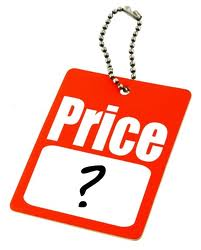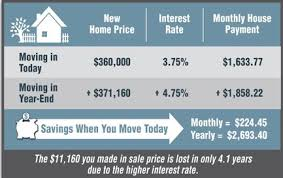# Difference between Price and Rates

##### Key Difference: A price refers to an amount of money that is fixed; generally in context to worth of a commodity in monetary terms, whereas rate is the measurement as a ratio to compare two different units.Price is generally used in context to monetary terms; it is the amount of money which determines the worth of a product or service. For example, when a consumer goes to buy a product, then the obvious question that comes into the mind of consumer is related to the cost of the commodity. The price many be expressed in written or may be asked to the shopkeeper.

The price is the amount that shopkeeper wants for the commodity. If the consumer likes the product and is ready to pay the price, he gives the amount of money equivalent to the price, and buys the commodity. In case, the customer is looking for some kind of discount, then he may ask the shopkeeper to lower the amount. It depends upon the shopkeeper to sell it on bargain price or not.

Some people often get confused with the words cost and value, and think that both are same. Value is quite different from cost; cost is actually the amount of money that is incurred in the manufacturing of the product, whereas the person may sell the product at more amount than cost, for the purpose of getting some profit. Then the cost becomes the price. Now days, the terms are being used interchangeably.Rate can be defined as a quantity measured as a ratio that is used to compare two different units. For example, the rate of speed of car can be expressed in miles per second. The quantity measured in this example is speed and is calculated by dividing the distance covered with the time taken to cover that distance. The result will be rate, indicating the distance covered per second.  Rate can also be used in context to measurement of a part with respect to a whole. For example, mortality rate, birth rate etc. Rate can also be a charge calculated in relation to a particular sum or quantity like the interest rates are measured.

However, when the differentiation of price and rate is to be made then, the contrast has to be in terms of amount of money. A price refers to an amount of money that is fixed, whereas a rate refers to an amount that is charged according to some period of time or with reference to some number of items. For example, if a tutor takes 500 dollars to teach students for five hours. We can define that the rate for the tuition is 100 dollars per hour.